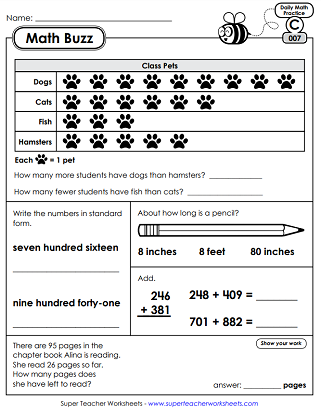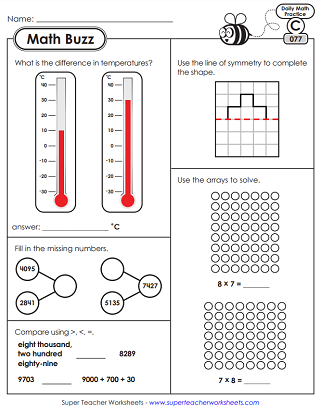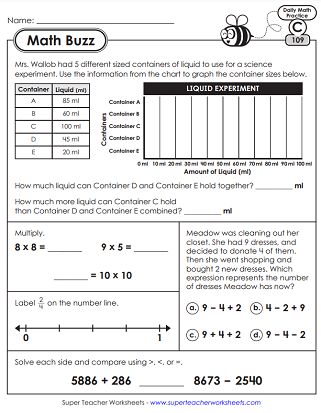# Daily Math Review Worksheets - Level C (3rd Grade)

Math Buzz is a series of 150 daily, spiraled math practice review worksheets for students. The third grade series includes common core-aligned skills that will help your students build and retain knowledge of math concepts throughout the school year.This file contains the first 5 days of daily math review for 3rd graders. (This file covers skills from the end of 2nd grade.) Skills include: 2-digit and 3-digit addition and subtraction, counting money, reading analog clocks, measurement with nonstandard units, polygons, and word problems.
Skills for this week include reading analog clocks, word problems, writing numbers in expanded form, reading a pictograph, measurement, dividing shapes into equal parts, and counting money.
On these worksheets, students will practice and review naming fraction parts, counting place value blocks, bar model addition and subtraction, expanded form, and measuring centimeters.
In week 4 of Math Buzz, students will practice measurement, naming 3D solid shapes, place value, dividing shapes into equal parts, and making a bar graph.
Within this set you'll find a variety of word problems, determining odd from even, telling time questions, geometry problems, and equal parts questions.
In the sixth week of Math Buzz, students will compare metric linear measurements, count money, divide rectangles into thirds, order numbers from greatest to least, use multiplication or repeated addition to solve an equal groups word problem.
Practice reading line plots, comparing numbers, comparing money amounts, number bonds, basic multiplication word problems, and rounding.
On these printable activities, your class will write repeated addition number sentences, complete addition/subtraction bar models, interpret a line plot, interpret a pictograph, and draw hands on an analog clock.
On these worksheets, students will be asked to practice basic multiplication with arrays, tell what fraction of a shape is shaded, add, subtract, compare 3-digit numbers, and solve a measurement word problem.
This daily review set has more patterns, clocks, and base-10 place value blocks. There are also questions about capacity and calendars.
In week eleven, we cover multiples, arrays, word problems, perimeter, and creating bar graphs.
On these printables, students will solve basic multiplication problems (0s, 1s, and 2s), write numbers in expanded form, look for right angles, and calculate elapsed time.
This file contains five worksheets. Skills covered include: liner measurement, input-output boxes (subtraction), perimeter of a trapezoid, and reading thermometers.
This set includes place value blocks, addition input/output boxes, fractions of a set, arrays, and linear measurement.
This one has more arrays, a multiplication bar model, polygon questions, analog clocks, a perimeter questions, and a bar graph.
Answer questions related to symmetry, number bonds, arrays, thermometers, and missing addends, and multi-step word problems.
On these daily math printables, your students can practice rounding on a number line, identifying polygons with right angles, telling time, and basic multiplication facts.
Review fractions on a number line (quarters), types of angles, multiples of a number, rounding with a number line, and perimeter.
On these sheets your class will calculate elapsed time, find missing factors, divide using models, and find shapes with parallel lines.
Here students will differentiate between open and closed shapes, solve an elapsed time problem, compare numbers, and find multiples of a number.
Count square units to find area of a square, solve a division problem using a graphic model, find missing numbers in 4-digit addition and subtraction problems, solve a multi-step word problem, and identify fractions on a number line.
With these worksheets, your class can solve an elapsed time problem using a number line, divide a trapezoid into equal parts, name fractional points on a number line, make a bar graph, and identify right angles.
Find the rule for the input/output table, tell how to solve a multi-step word problem, complete operations with parenthesis, write mixed numbers on a number line, and multiply to find the area of a square.
Week twenty-four of our daily math review includes input and output tables, area, perimeter, and some multiplication and division facts.
Within this set of daily math review you will find
3-digit by 3-digit subtraction, multiplication problems, word problems, weight, and division problems.
On these worksheets, your students will be asked to find the area and perimeter of a rectangle, find the capacity of two graduated cylinders, and complete some input and output boxes.
On these printables, students will solve basic multiplication and division problems, find equivalent fractions, and compare some numbers and fractions.
On these daily math printables, your students will solve some division and multiplication problems, find the area of some rectangles, complete the in and out boxes, and compare numbers.
In week 29 of Math Buzz, students will practice filling in the missing numbers of math problems, compare numbers, and find the area of some rectangles.
This daily review set has more input and output tables, number line fractions, area problems, and more!
More Math Daily Review

We have daily math review concepts for all elementary grades.

## Sample Worksheet ImagesMy Account
Site Information